Fox, Jacob

Complete bipartite subgraphs of perfect graphs ★★

Author(s): Fox

Problem   Letbe a perfect graph onvertices. Is it true that eitheror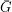contains a complete bipartite subgraph with bipartition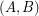so that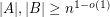?

Keywords: perfect graph

Long rainbow arithmetic progressions ★★

Author(s): Fox; Jungic; Mahdian; Nesetril; Radoicic

Forletdenote the minimal number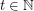such that there is a rainbowin every equinumerous-coloring of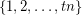for every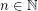Conjecture   For all,.

Keywords: arithmetic progression; rainbow